2019-08-19 17:56:03 weixin_38742571 阅读数 836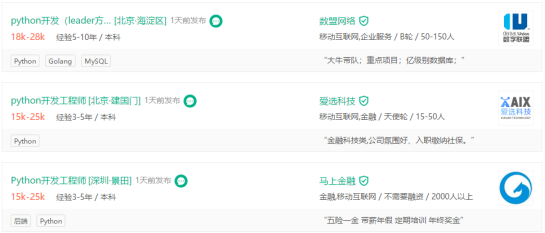Python工程师月薪高达15K以上，那Python工程师主要做什么？Python工程师未来前景如何？Python编程 行业分析与课程简介

《21天通关Python》视频课程以畅销图书为教材，由图书作者李刚亲自操刀讲解；上手门槛低，可作为0基础掌握Python教材；书籍+线上复合型学习场景特别适合Python小白学习！

（含图书邮寄+视频教程+社群答疑+导师带队）2018-04-18 21:01:12 xyisv 阅读数 4682

python机器学习

python机器学习只适合做小规模的算法和简单神经网络，适合入门新手学习，由于Python的效率极低，一般前沿研究中不使用Python进行大数据学习。因此下面很多算法是基于Python模块编写的机器学习代码。

特征选择算法

2019-07-02 09:19:03 cp_oldy 阅读数 1065

1. Anomaly Detection 异常检测

novelty, exception, outlier

• 异常类不止1类
• 异常类样本难收集

2. Attack & Defense 攻击和防御

Motivation:

• labs->real world
• robust
• malicious attack

L-infinity 适合图像攻击
$d(x^0, x') = ||x^0-x'||_{\infty} = \max{\{\Delta x_1, \Delta x_2, \Delta x_3, \cdots\}}$

L2范数不适合图像攻击的小例子，每个像素修改一点和一个像素修改很多，L2范数变化完全一样，但是视觉效果相差很大。

物理世界中的攻击需要考虑更多

• 有效性（多角度有效）
• 鲁棒性（不是像素，是色块，可被相机捕获）
• 可行性（可打印颜色）；

Defense 防御

weight regularization, dropout, model ensemble对对抗攻击不能够完全防住。

防的两种策略：

• 被动防御： 异常检测的特例。
• 主动防御： 训练时加入防御。

被动防御

1. filter
2. scale
这些策略，不会对正常图片有很大的影响；对攻击图像有效果。

3. Explainable ML 可解释学习

遇到错误怎么办？

unexplainable ML：暴力调参
explainable ML：根据解释改变模型

local explanation：

object X $\Rightarrow$ Components: {$x_1, ..., x_n, ...$}

$|\frac{\partial y_k}{\partial x_n}|$，得到

攻击解释性？！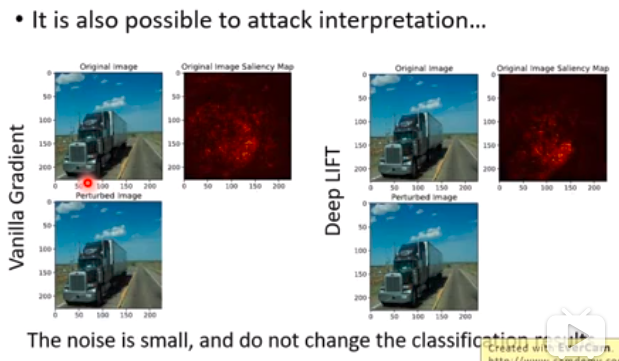global explanation：

Question: what do you think a cat look like?

Activation Maximization激活最大化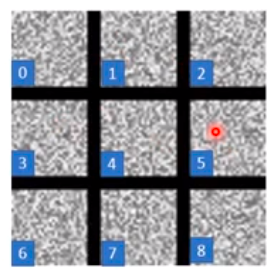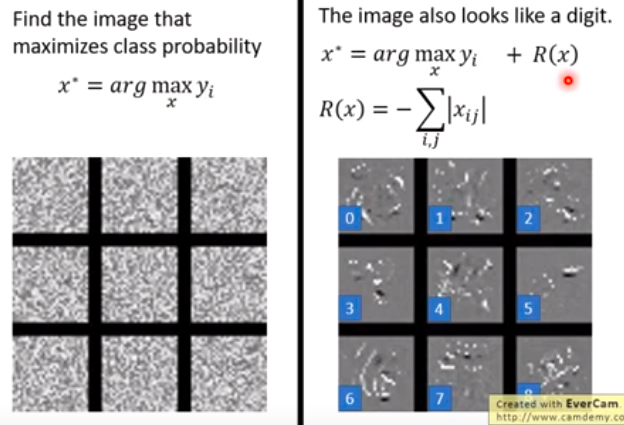用可解释模型去解释不可解释模型

• 显然，线性模型是可解释的。问题：线性模型并不能够模拟神经网络。

• 用决策树来解释神经网络。问题：我们不希望树太大。

4. 终身学习

Life Long Learning (LLL)
continuous learning, never ending learning, incremental learning

• knowledge retention 知识保持：不固执
• knowledge transfer 知识迁移
• model expansion 模型扩展：有效率扩展

Catastrophic Forgetting 灾难性遗忘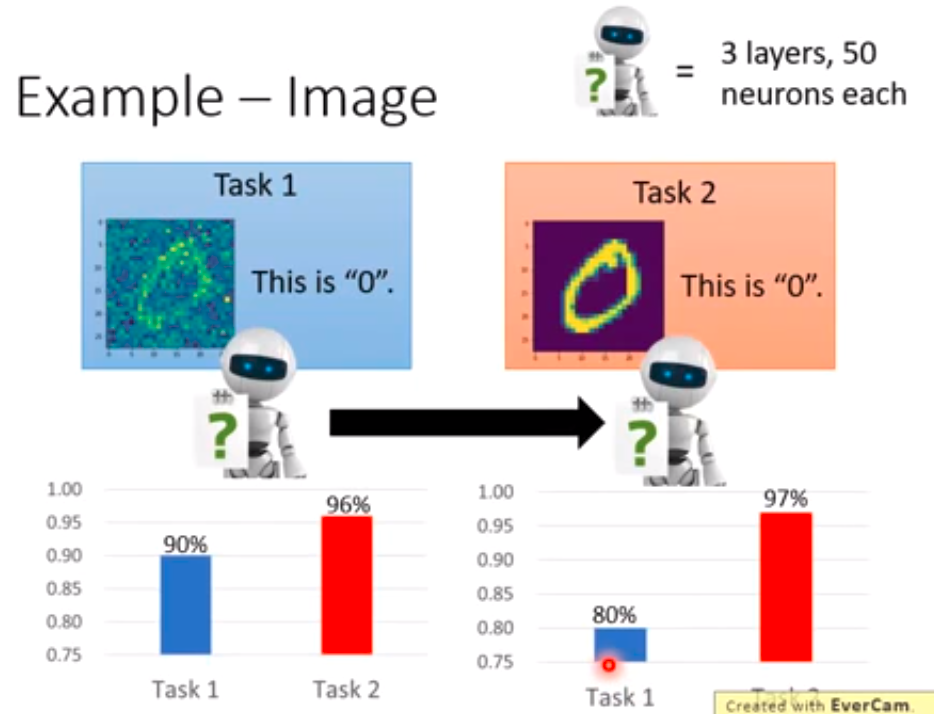LLL的上界是多任务学习！！！

elastic weight cosolidation (EWC）
basic idea: 和旧知识相关的权重不修改，只修改不重要的权重。每个权重有一个守护$b_i$$b_i$可以是权重的二次微分。

life long learning和transfer learning的区别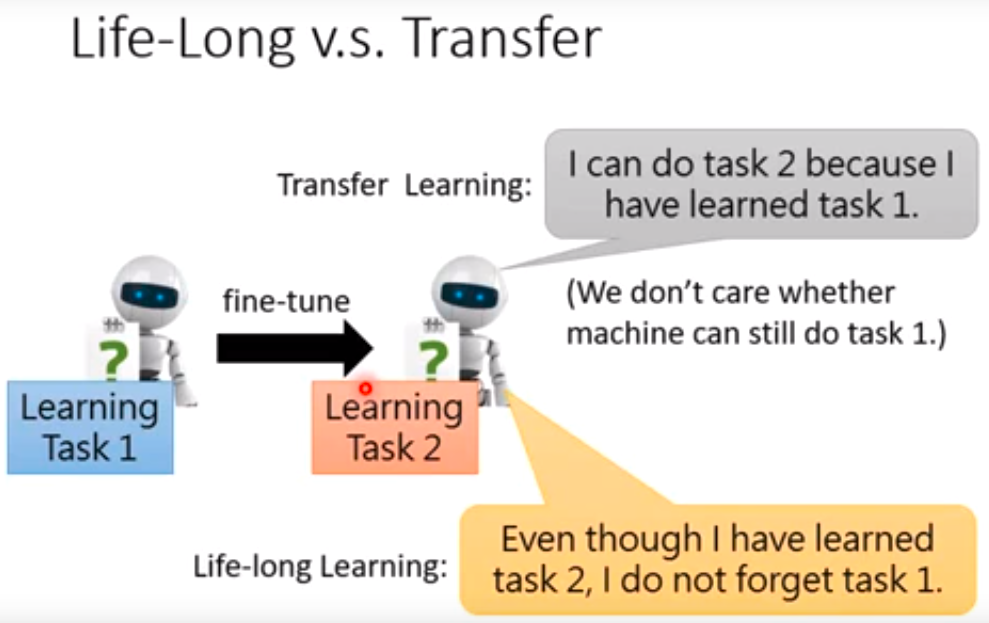5. 元学习

f(x)=‘cat’

F(X)=f*

1. 收集训练任务和测试任务（验证集）
2. 评估F的方式
Defining the goodness of a function F
$L(F) = \sum_{n=1}^{N} l^n$
把N个任务的loss相加。
3. 选择合适的F*

MAML和pre-training

MAML是要找到一个初始化的参数$\phi$，经过训练后能够找到最好的$\theta^n$对每个任务效果好。关注的好的初始点，重点在训练之后
pre-training是要找到一个初始化参数$\phi$对所有任务效果好。关注的是现在的效果，不继续训练

MAML、Reptile、Pre-train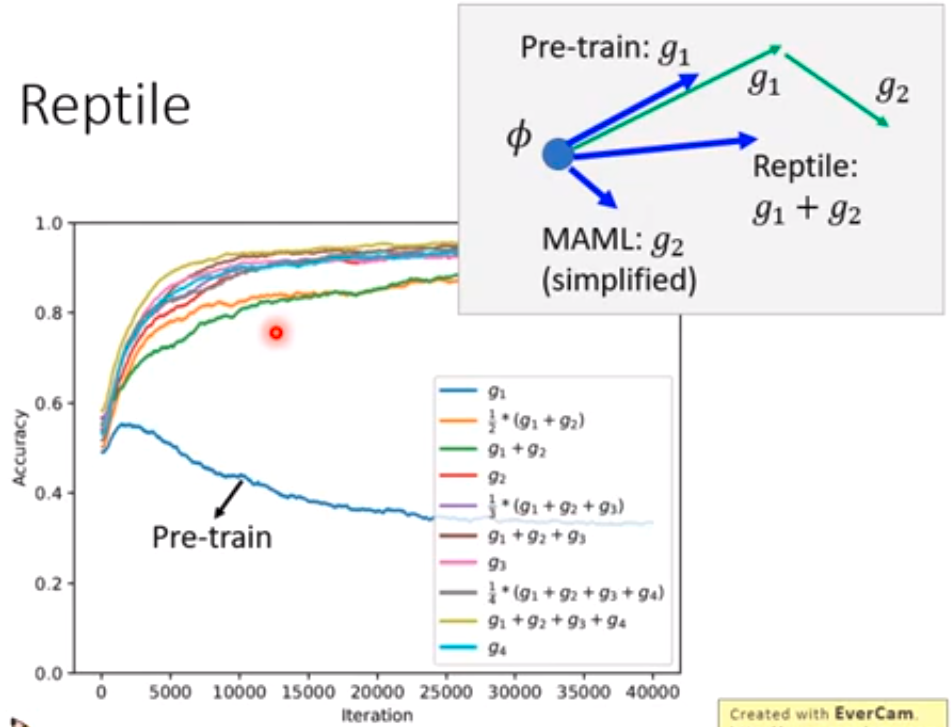2018-02-22 21:49:44 u011626960 阅读数 30872017-04-14 22:47:34 hicheney 阅读数 2015

机器学习概述

@(机器学习)

机器学习基本概念

1、机器学习算法类型

根据训练数据提供的信息以及反馈方式的不同，可以分为：
* 有监督学习(Supervised Learning)：每组训练数据都有一个明确的标签和结果，利用这些已知的数据来学习模型的参数，使得模型预测的目标标签和真实标签尽可能接近。涉及的应用：预测房屋的价格，股票的涨停，垃圾邮件检测。神经网络也是一种监督学习的方式。常见算法有逻辑回归(Logistic Regression)和反向传递神经网络(Back Propagation Neural Network)。根据目标标签的类型不同，又可以分为：
* 回归(Regression)问题：目标标签y是连续值（实数或连续整数）,f(x)的输出也是连续值。
* 分类(Classification)问题：目标标签y是离散的类别（符号）。在此问题中，通过学习得到的决策函数也叫分类器• 无监督学习(Unsupervised Learning)：学习的数据不包含目标标签，需要学习算法自动学习到一些有价值的信息。一个典型的问题就是聚类，常见算法包括Apriori算法以及k-Means算法。• 半监督学习(Semi-supervised Learning)：少量有目标标签的样本和大量没有目标标签的样本。先试图对未标识数据进行建模，在此基础上对标识的数据进行预测，如图论推理算法或者拉普拉斯支持向量机等。• 强化学习(Reinforcement Learning)：输入数据直接反馈到模型，模型必须立刻做出调整。也就是常说的从经验中总结提升。常见的应用场景包括动态系统以及机器人控制等，Google的AlphaGo就是运用了这种学习方式。算法本身会总结失败和成功的经验来达到很高的成功率。常见算法包括Q-Learning以及时间差学习（Temporal difference learning）。• 遗传算法(Genetic Algorithm)：模拟进化理论，适者生存不适者淘汰，通过淘汰机制选择最优化的模型。在企业数据应用的场景下， 人们最常用的可能就是监督式学习和非监督式学习的模型。 在图像识别等领域，由于存在大量的非标识的数据和少量的可标识数据， 目前半监督式学习是一个很热的话题。 而强化学习更多的应用在机器人控制及其他需要进行系统控制的领域。

2、风险函数与损失函数

我们需要建立一些准则来衡量决策函数的好坏，一般是定义一个损失函数L(y,f(x,θ))$L(y,f(x,\theta))$，然后在所有的训练样本上来评价决策函数的风险。

损失函数

给定一个实例(x,y)$(x,y)$，真实目标是y$y$，机器学习模型预测的结果为f(x,θ)$f(x,\theta)$，损失函数就是用来估量模型的预测值和真实值的不一致程度，它是一个非负实值函数，损失函数越小，模型的鲁棒性就越好。损失函数是经验风险函数的核心部分，也是结构风险函数的重要组成部分。

* 0-1损失函数：0-1损失函数（0-1 loss function）是

L(y,f(x,θ))=={01if y=f(x,θ)if yf(x,θ)I(yf(x,θ))

* 平方损失函数：通常为线性回归的损失函数。
L(y,y^)=(yf(x,θ))2

最小二乘法和梯度下降法都是通过求导来求损失函数的最小值。
* 最小二乘法：直接对L(y,f(x,θ))$L(y,f(x,\theta))$求导找出全局最小，是非迭代法；
* 梯度下降法：迭代法，先给定一个，然后向下降最快的方向调整，在若干次迭代之后找到局部最小。其缺点是到最小点的时候收敛速度变慢，并且对初始点的选择极为敏感。
* 交叉熵损失函数：对于分类问题，预测目标为y$y$为离散的类别，模型输出f(x,θ)$f(x,\theta)$为每个类的条件概率。
假设y{1,...,C}$y \in \{1,...,C\}$，模型预测的第i$i$个类的条件概率P(y=ix)=fi(x,θ)$P(y=i \mid x)=f_i(x,\theta)$，则f(x,θ)$f(x,\theta)$满足
fi(x,θ)[0,1]i=1Cfi(x,θ)=1

f_y(x,\theta)可以看做真实类别y$y$的似然函数。参数可以用最大似然估计来优化。考虑到计算问题，我们经常使用最小化负对数似然，也就是负对数似然损失函数。
L(y,f(x,θ))=logfy(x,θ)

如果我们用one-hot向量y$y$来表示目标类别c$c$，其中只有yc=1$y_c=1$，其余的向量元素都为0.
负对数似然函数可以写为：
L(y,f(x,θ))=i=1Cyilogfi(x,θ)

yi$y_i$也可以看成是真实类别的分布，这样上面的公式恰好是交叉熵的形式，因此，负对数似然函数也常叫做交叉熵损失函数。
* Hinge损失函数：对于两类分类问题，假设y$y$f(x,θ)$f(x,\theta)$的取值为{1,+1}$\{-1,+1\}$。Hinge损失函数的定义如下：
L(y,f(x,θ))=max(0,1yf(x,θ))=|1yf(x,θ)|+.

风险函数

• 经验风险Remp(θ)$R_{emp}(\theta)$，在已知的训练样本（经验数据）上计算得来。用对参数θ$\theta$求经验风险来逐渐逼近理想的期望风险的最小值，这一原则我们称为经验风险最小化原则
• 结构风险Rstruct(θ)$R_{struct}(\theta)$，在经验风险最小化的原则上加上参数的正则化，叫做结构风险最小化原则

3、常见的基本概念

数据

所有能由计算机程序处理的对象的总称，可以是数字、字母和符号等。

特征

在现实世界中，原始数据通常并不都是以连续变量或离散变量的形式存在的，我们首先需要抽取出一些可以表征这些数据的数值型特征，这些数值型特征可以表示为向量形式，也称为特征向量。

参数与超参数

需要通过学习算法学习得来的就是参数。超参数是参数的参数。

特征学习

如何自动地学习有效的特征也成为机器学习中一个重要的研究内容，也就是特征学习，也叫表示学习。特征学习分成两种，一种是特征选择，是在很多特征集合选取有效的子集；另一种是特征提取，是构造一个新的特征空间，并将原始特征投影到新的空间中。

样本

按照一定的抽样规则从从全部数据中取出的一部分数据，是实际观测到的数据。

训练集和测试集

一组样本的集合就称为数据集。为了检验机器学习算法的好坏，一般将数据集分成两部分：训练集和测试集。训练集用来进行模型学习，测试集用来进行模型验证。

正例和负例

对于两类分类问题，常用正例和负例来分别表示属于不同类别的样本。

判别函数

经过特征提取后，一个样本可以表示为k$k$维特征空间中的一个点。为了对这些点进行分类，需要一些超平面来将这个特征空间分成一些互不重叠的子区域，使得不同类别的点分布在不同的子区域中，这些超平面就是判别界面。